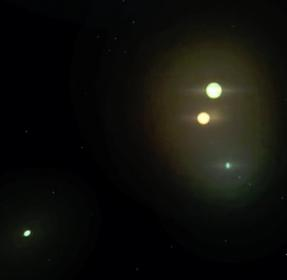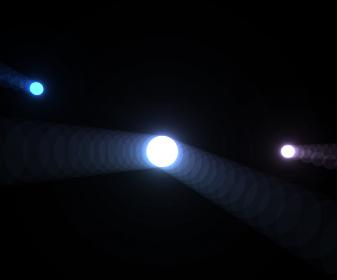# Help with coordinates problem.

Hi!
Im trying to apply this radial shader in multiples spheres, but unsuccessfully.
the problem is related with screen coordinates. The shader only works if the sphere is in the center of the screen, just like show this pictures:

but if the center is not align to camera, this is what happen:

as you see, you can see the samples compute in the fragment shader:

``````#version 150

in vec2 varyingtexcoord;
uniform sampler2DRect tex0;
uniform sampler2DRect depth;

uniform vec3 ligthPos;
float exposure = 0.19;
float decay = 0.9;
float density = 2.0;
float weight = 1.0;
int samples = 25;

out vec4 fragColor;
const int MAX_SAMPLES = 100;
void main()
{
//        float sampleDepth = texture(depth, varyingtexcoord).r;
//
//        vec4 H = vec4 (varyingtexcoord.x *2 -1, (1- varyingtexcoord.y)*2 -1, sampleDepth, 1);
//
//    vec4 = mult(H, gl_ViewProjectionMatrix);

vec2 texCoord = varyingtexcoord;
vec2 deltaTextCoord = texCoord - ligthPos.xy;
deltaTextCoord *= 1.0 / float(samples) * density;
vec4 color = texture(tex0, texCoord);
float illuminationDecay = .6;
for(int i=0; i < MAX_SAMPLES; i++)
{
if(i == samples){
break;
}
texCoord -= deltaTextCoord;
vec4 sample = texture(tex0, texCoord);
sample *= illuminationDecay * weight;
color += sample;
illuminationDecay *= decay;
}
fragColor = color * exposure;
}
``````

the shader has an uniform input called ligthPos. I discovered a function in openframeworks called cam.worldToScreen(); if i send the result of this function to the shader, then the sphere is moving in all over the screen without problem. The problem is that i want more than just one sphere, so the logic of this shader is not correct. What you suggest? i want to apply this radial blur (god ray style) to a undefine number of spheres and other shapes. this is an example:what do you think? i notice that the post processing shader (in the first pass i render the geometry, then i pass that buffer to a other fbo with this shader) affects the all screen, but i need a method to fix this. please, i need suggestions. im new in shader, so a didactic example would be great. thanks! O.

Well, ignoring optimizations…

Since you’re currently passing in one light to generate one sphere, the simplest thing to do is pass in an array of lights to generate N spheres.

Alternatively, you could multi-pass this (i.e. do one draw+shader pass per light), but that gets expensive after a point.

Also keep in mind that if the extent of the sphere’s isn’t a significant fraction of the area on the screen, you can save quite a bit of fill by limiting the bounds of the quads you’re rendering.

thanks. i actually using an array of locations for each lightPos. But this is my problem now. I understand that this kind of post processing shader affect all pixels of the texture that i send it. So, look whats happens:notice how the effect is computed well for every iteration, but i cant avoid those others samples generated for each iteration. this is the shader:

``````#version 150

in vec2 varyingtexcoord;
uniform sampler2DRect tex0;

uniform int size;

float exposure = 0.79;
float decay = 0.9;
float density = .9;
float weight = .1;
int samples = 25;

out vec4 fragColor;
const int MAX_SAMPLES = 25;
const int N = 3;
uniform vec2 ligthPos [N];

int a = 1;

vec4 halo(vec2 pos){

float illuminationDecay = 1.2;
vec2 texCoord = varyingtexcoord;
vec2 current = pos.xy;
vec2  deltaTextCoord = texCoord - current;

deltaTextCoord *= 1.0 / float(samples) * density;
vec4 color = texture(tex0, texCoord);

for(int i=0; i < MAX_SAMPLES; i++){
texCoord -= deltaTextCoord;
vec4 sample = texture(tex0, texCoord);
sample *= illuminationDecay * weight;
color += sample;
illuminationDecay *= decay;
}
return color;
}

void main(){
vec2 uv = varyingtexcoord;
vec4 color = texture(tex0, uv);
vec4 accum = vec4(0.0);
for(int e = 0; e < N;e++){
vec2 current =ligthPos[e];
accum += halo(current);
}
fragColor = (accum) * exposure;
}
``````